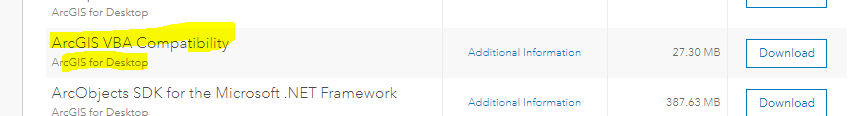# VB Script is not working

623
8
02-20-2018 10:08 AMNew Contributor III

Hi there,

Can anyone please have a look in the script below;

If[AssetCondition] = "1 - NEW" Then
[CONDITION] = "1"
ElseIf[AssetCondition] = "2 - GOOD" Then
[CONDITION] = "2"
ElseIf[AssetCondition] = "3 - POOR" Then
[CONDITION] = "3"
ElseIf[AssetCondition] = "4 - UNSERVICEABLE" Then
[CONDITION] = "4"
Else [CONDITION] = "NULL"
End If

It's not working. Any assistance will be highly appreciated, thanks

1 Solution

Accepted SolutionsbyMVP Honored Contributor

I have several comments.  If you are using the Field Calculator you cannot set a field value in VB Script with an expression like:

[CONDITION] = "1"

You can only set a field value by doing a calculation on that field.  Field names in brackets are read only.

You need to make the output of the calculation a variable name, not a field name.  I use the variable Output in all my Pre-Logic VB calculations. You need a space after keywords like If and ElseIf.  Else should be by itself in a multiline If Else Then expression.  Null is not quoted if you want a real Null value.

Here is the Field Calculator set up you need to use while doing the calculation on the CONDITION field:

Parser: VB Script

Show Codeblock checked

Pre-Logic Script Code:

If [AssetCondition] = "1 - NEW" Then
Output = "1"
ElseIf [AssetCondition] = "2 - GOOD" Then
Output = "2"
ElseIf [AssetCondition] = "3 - POOR" Then
Output = "3"
ElseIf [AssetCondition] = "4 - UNSERVICEABLE" Then
Output = "4"
Else
Output = Null
End If

CONDITION =

Output

8 RepliesbyMVP Esteemed Contributor

Not much on VB script these days, but I'm pretty sure you need indents.  See If ... Then...Else - VBScript - SS64.com

You might get a better response if this were posted in something other than the Python section

That should just about do it....byMVP Esteemed Contributor

What isn't working?  An error message or just unexpected results?  If the former, what is the error message?  If the latter, what are you expecting vs what you are seeing?byMVP Esteemed Contributor

You don't mention the software or version you are tryin gto work with, but maybe you need the VBA Compatibility?  I had to go back to the 10.4.1 download (on myesri) to find the last available version for Desktop.byMVP Regular Contributor

Indeed, the newer versions of ArcGIS don't support VBA. You should really convert your VBA logic to Python.

``````if !AssetCondition! == "1 - NEW":
!CCONDITION! = "1"
elif !AssetCondition! ==  "2 - GOOD":
!CCONDITION! = "2"
elif !AssetCondition! ==  "3 - POOR":
!CCONDITION! = "3"
elif !AssetCondition! ==  "4 - UNSERVICEABLE":
!CCONDITION! = "4"
else:
!CONDITION! = None‍‍‍‍‍‍‍‍‍‍``````byMVP Honored Contributor

This Python expression won't work in the field calculator.  You need to declare it indented under a def method you have named and you need to have passed all of the field values in through that method.  Like VB Script, you can only set a field value by doing a calculation on a field and returning a value from your Pre-Logic Script code, not by using an expression internal to the calculation like:

!Condition! = "1"

So in Python it would be:

Parser: Python

Show Codeblock checked

PreLogic Script Code:

``````def setCondition(assetCondition):
if assetCondition == "1 - NEW":
return "1"
elif assetCondition ==  "2 - GOOD":
return "2"
elif assetCondition ==  "3 - POOR":
return "3"
elif assetCondition ==  "4 - UNSERVICEABLE":
return "4"
else:
return None‍‍‍‍‍‍‍‍‍‍‍‍‍‍‍‍‍‍‍‍‍‍‍‍‍‍‍‍‍‍‍‍‍``````

Condition =

setCondition(!AssetCondition!)byMVP Regular Contributor

Good point, Richard Fairhurst. My intention was just to show a simple like for like comparison of the VB code with Python. Thanks for clarifying and making a working example for colasuk‌.byMVP Honored Contributor

I have several comments.  If you are using the Field Calculator you cannot set a field value in VB Script with an expression like:

[CONDITION] = "1"

You can only set a field value by doing a calculation on that field.  Field names in brackets are read only.

You need to make the output of the calculation a variable name, not a field name.  I use the variable Output in all my Pre-Logic VB calculations. You need a space after keywords like If and ElseIf.  Else should be by itself in a multiline If Else Then expression.  Null is not quoted if you want a real Null value.

Here is the Field Calculator set up you need to use while doing the calculation on the CONDITION field:

Parser: VB Script

Show Codeblock checked

Pre-Logic Script Code:

If [AssetCondition] = "1 - NEW" Then
Output = "1"
ElseIf [AssetCondition] = "2 - GOOD" Then
Output = "2"
ElseIf [AssetCondition] = "3 - POOR" Then
Output = "3"
ElseIf [AssetCondition] = "4 - UNSERVICEABLE" Then
Output = "4"
Else
Output = Null
End If

CONDITION =

OutputNew Contributor III

That's absolutely wonderful from you Richard. It worked very well for me. I appreciate your time and effort.